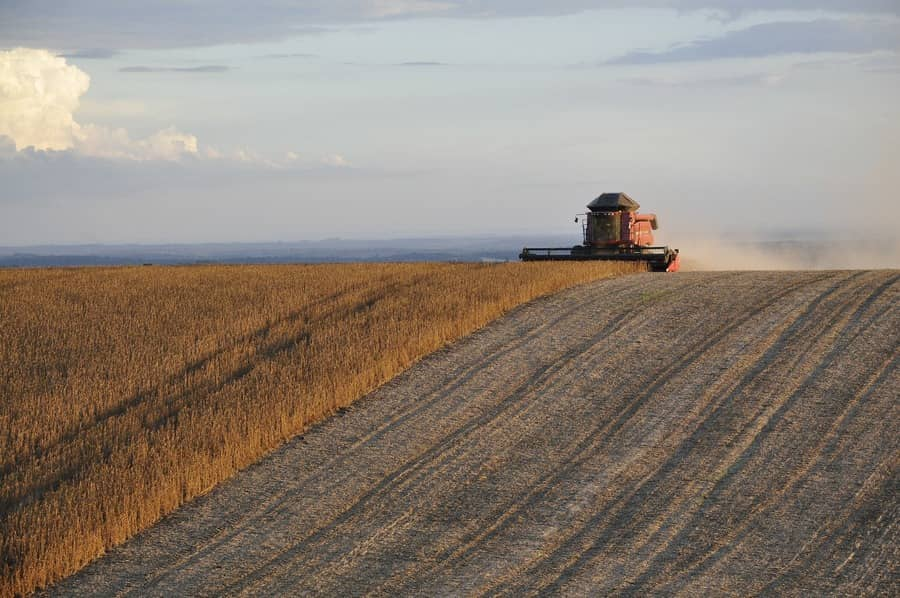# Brazilian soybean harvest advances to the final stretch, but rain hinders again

95Porto Alegre, May 2, 2022 – The harvest of the new Brazilian soybean season has advanced to the final stretch in the country. Despite this, higher moisture once again prevented a better advance of the machines in the South Region, where work is still delayed. This fact keeps the Brazilian rhythm below the five-year average, although the harvest has already ended in several growing states.

According to a survey carried out by SAFRAS & Mercado, until April 29, 93.8% were reaped from a total planted area of 40.78 million hectares, equivalent to approximately 38.261 million hectares. In the previous week, the percentage was 91.4%. In the same period last year, the percentage was 95.1%. The five-year average for the period is 96.2%.

In Rio Grande do Sul, out of a total sown area of 6.3 million hectares, 69% were reaped, or approximately 4.347 million hectares. In the previous week, the percentage was 57%. In the same period last year, the percentage was 81%. The five-year average for the period is 90%.

In Paraná, out of a total area of 5.65 million hectares, 99% were reaped, or the equivalent to approximately 5.594 million hectares. In the previous week, the percentage was 99%. In the same period last year, the percentage was 100%, while the five-year average for the period is 99.6%.

In Mato Grosso, out of a total sown area of 10.9 million hectares, 100% were reaped. The week earlier, the percentage was 100%. In the same period last year, the percentage was 100%. The five-year average for the period is 100%.

In Mato Grosso do Sul, out of a total sown area of 3.8 million hectares, 100% were reaped. The week earlier, the percentage was 100%. In the same period as the previous week, the percentage was 100%, while the five-year average for the period is 100%.

In Goiás, out of a total sown area of 3.94 million hectares, 100% were reaped. The week earlier, the percentage was 100%. In the same period last year, the percentage was 100%. The five-year average for the period is 100%.

In São Paulo, out of a total sown area of 1.22 million hectares, 99% were reaped, or approximately 1.208 million hectares. In the previous week, the percentage was 99%. In the same period last year, the percentage was 100%, while the five-year average for the period is 100%.

In Minas Gerais, out of a total area estimated at 2 million hectares, 100% were reaped. In the previous week, the percentage was 99%. In the same period last year, the percentage was 100%. The five-year average for the period is 99.6%.

In Bahia, out of a total sown area of 1.78 million hectares, 99% were reaped, or approximately 1.762 million hectares. In the previous week, the percentage was 99%. In the same period last year, the percentage was 95%. The five-year average for the period is 87.4%.

Weather maps point to a period of greater moisture in the South Region between April 29 and May 5, which may again hinder the progress of machines in Rio Grande do Sul and Santa Catarina. Other growing states in the country must receive little or no moisture in the period, except for Pará.